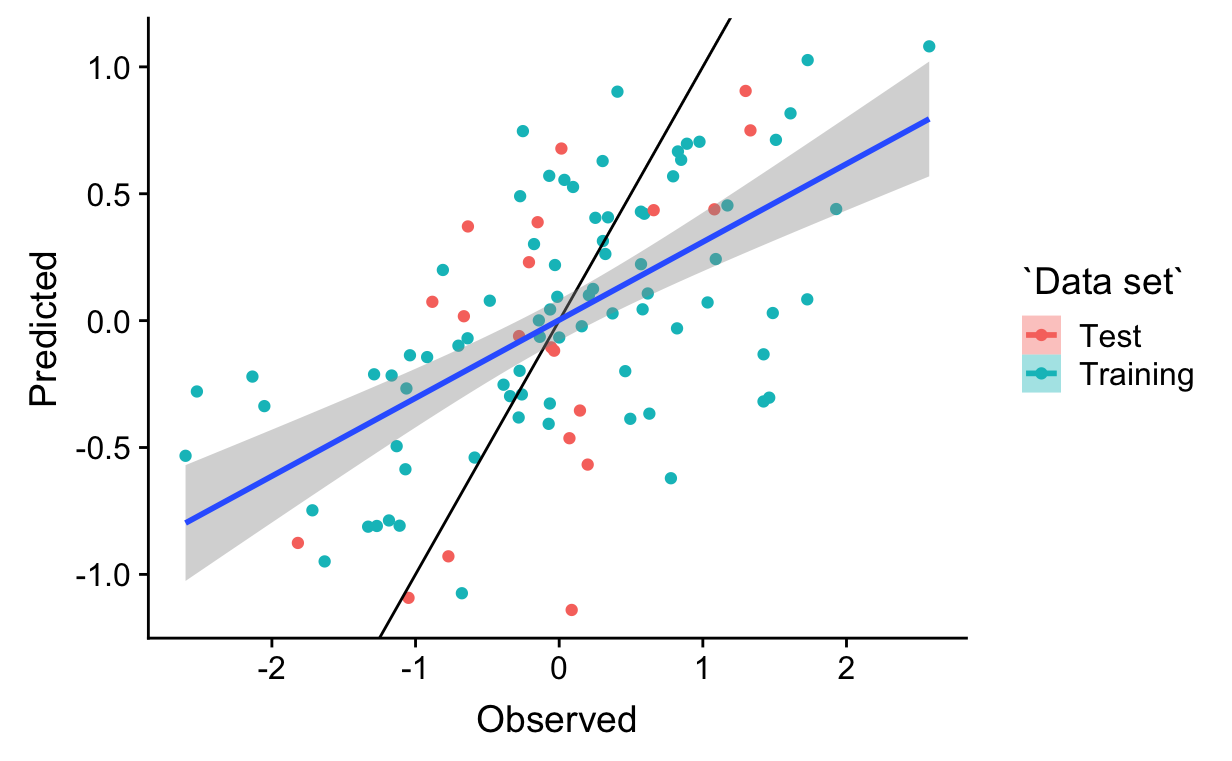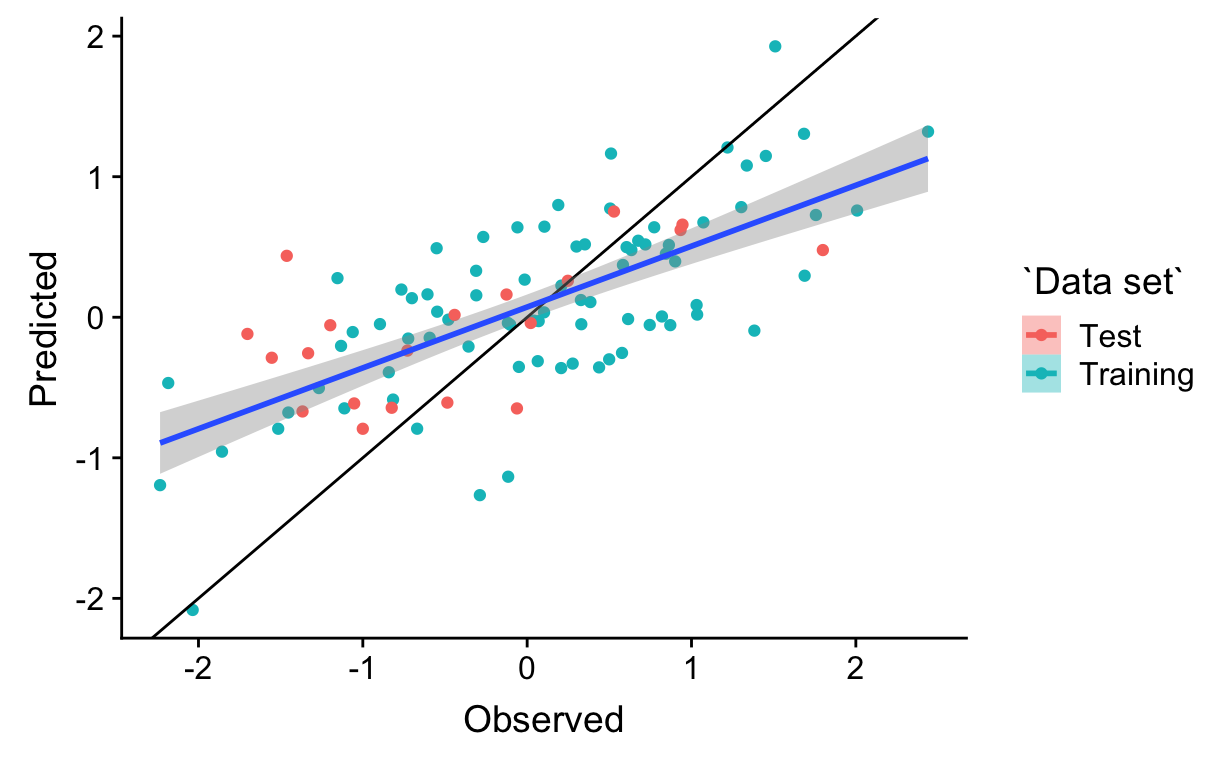The problem of identifying covariates that should be included in a regression has been studied for a long time. More than two decades ago, Rob Tibshirani introduced the Lasso to let the data tell us which covariates to include. If you want the details, including how the Lasso has been generalized, refer to the Wikipedia page. For our purposes, it’s enough for you to know that for linear regression fit by least squares, the Lasso simply includes a constraint on the regression coefficients.

## What is the Lasso?

To make that constraint clear, let’s write out explicitly what the least squares criterion is:

$\mbox{min}_{\beta}\left\{\frac{1}{N}\sum_i\left(y_i - (\beta_0 + \sum_j\beta_jx_{ij})\right)^2\right\}$

In words this simply means that we find the set of $$\beta$$s that minimize the difference between our predictions and the observed data. The Lasso does that too, but it adds a constraint on the set of $$\beta$$s, namely

$\sum_j |\beta_j| < \lambda \quad ,$

where $$\lambda$$ is a parameter that determines the maximum influence of all covariates on the response variable when all of the individual influences are combined. To incorporate the constraint into the algorithm we use this criterion:

$\mbox{min}_{\beta}\left\{\frac{1}{N}\sum_i\left(y_i - (\beta_0 + \sum_j\beta_jx_{ij})\right)^2 + \lambda\sum_j|\beta_j|\right\}$

In a simple least squares regression we don’t care how big any of the $$\beta$$s are individually and we don’t care about how big their overall magnitude is. In using the Lasso we are making the assumption that not only are we unwilling to accept the idea that any individual $$\beta$$ is large, but we are also unwilling for any combination of them to be large either. The effect of this, as we’ll see is not only to limit the magnitude of individual regression coefficients, but also to keep some of them close to zero.

## Trying out the Lasso

As usual, our first step is to regenerate the data we’ve been playing with.

library(tidyverse)
library(reshape2)
library(ggplot2)
library(cowplot)
library(mvtnorm)
library(corrplot)

rm(list = ls())
## intetcept
##
beta0 <- 1.0
## regression coefficients
##
beta <- c(1.0, -1.0, 1.0, 0.0, 0.0, 0.0, 0.0, 0.0, 0.0)
## pattern of correlation matrix, all non-zero entries are set to saem
## correlation, covariance matrix caldulated from individual variances and a
## single association parameter governing the non-zero correlation coefficients
##
## Note: Not just any pattern will work here. The correlation matrix and
## covariance matrix generated from this pattern must be positive definite.
## If you change this pattern, you may get an error when you try to generate
## data with a non-zero association parameter.
##
Rho <- matrix(nrow = 9, ncol = , byrow = TRUE,
data = c(1,0,1,0,1,0,1,0,1,
0,1,0,1,0,1,0,1,0,
1,0,1,0,1,0,1,0,1,
0,1,0,1,0,1,0,1,0,
1,0,1,0,1,0,1,0,1,
0,1,0,1,0,1,0,1,0,
1,0,1,0,1,0,1,0,1,
0,1,0,1,0,1,0,1,0,
1,0,1,0,1,0,1,0,1
))
## vector of standard deviations for covariates
##
sigma <- rep(1, 9)

## construct a covariance matrix from the pattern, standard deviations, and
## one parameter in [-1,1] that governs the magnitude of non-zero correlation
## coefficients
##
## Rho - the pattern of associations
## sigma - the vector of standard deviations
## rho - the association parameter
##
construct_Sigma <- function(Rho, sigma, rho) {
## get the correlation matris
##
Rho <- Rho*rho
for (i in 1:ncol(Rho)) {
Rho[i,i] <- 1.0
}
## notice the use of matrix multiplication
##
Sigma <- diag(sigma) %*% Rho %*% diag(sigma)
return(Sigma)
}

## set the random number seed manually so that every run of the code will
## produce the same numbers
##
set.seed(1234)

n_samp <- 100
cov_str <- rmvnorm(n_samp,
mean = rep(0, nrow(Rho)),
sigma = construct_Sigma(Rho, sigma, 0.8))

resid <- rep(2.0, n_samp)

y_str <- rnorm(nrow(cov_str), mean = beta0 + cov_str %*% beta, sd = resid)
dat_1 <- data.frame(y_str, cov_str, rep("Strong", length(y_str)))

cov_str <- rmvnorm(n_samp,
mean = rep(0, nrow(Rho)),
sigma = construct_Sigma(Rho, sigma, 0.8))
y_str <- rnorm(nrow(cov_str), mean = beta0 + cov_str %*% beta, sd = resid)
dat_2 <- data.frame(y_str, cov_str, rep("Strong", length(y_str)))

column_names <- c("y", paste("x", seq(1, length(beta)), sep = ""), "Scenario")
colnames(dat_1) <- column_names
colnames(dat_2) <- column_names

## saving results in scale allows me to use them later for prediction with
## new data
##
scale_1 <- lapply(dat_1[, 1:10], scale)
scale_2 <- lapply(dat_2[, 1:10], scale)

## when assigning the same scaling to a data frame, the scaling attributes
## are lost
##
dat_1[, 1:10] <- lapply(dat_1[, 1:10], scale)
dat_2[, 1:10] <- lapply(dat_2[, 1:10], scale)

OK. Now that we have the data, let’s try the lasso and see what we get.1

### Fitting the lasso

The first thing we have to do is to find a value for $$\lambda$$. A good approach is to cv.glmnet(), which will split the data set into test and training data set and use cross-validation to identify the best value.2 Since we’re going to do this for both data sets, we start by writing a function that will do it all for us. In this function, I holdout some of the data, by default 20 percent, to illustrate how well the model performs on within sample predictions.

library(glmnet)

lasso <- function(dat, title, holdout = 0.2) {
x_vars <- model.matrix(y ~ x1 + x2 + x3 + x4 + x5 + x6 + x7 + x8 + x9,
data = dat)[, -1]
y_var <- dat$y ## select the training set (a random sample from 50% of the rows) ## train <- sample(1:nrow(x_vars), nrow(x_vars)*(1.0 - holdout)) ## construct the text set ## test <- setdiff(1:nrow(x_vars), train) ## use a series of different lambda's that cv.glmnet() picks to identify ## the best one ## cv_output <- cv.glmnet(x_vars[train, ], y_var[train]) ## now predict values for the test set and the training set so that we can ## compare observations with predictions for both of them ## predict_train <- predict(cv_output, x_vars[train, ], s = "lambda.min") predict_train_mat <- cbind(y_var[train], predict_train, "Training") if (holdout > 0) { predict_test <- predict(cv_output, x_vars[test, ], s = "lambda.min") predict_test_mat <- cbind(y_var[test], predict_test, "Test") predict <- rbind(predict_train_mat, predict_test_mat) } else { predict <- predict_train_mat } colnames(predict) <- c("Observed", "Predicted", "Data set") predict <- as.data.frame(predict) predict$Observed <- as.numeric(as.character(predict$Observed)) predict$Predicted <- as.numeric(as.character(predict\$Predicted))

if (holdout > 0) {
p <- ggplot(predict, aes(x = Observed, y = Predicted,
color = Data set, fill = Data set))
} else {
p <- ggplot(predict, aes(x = Observed, y = Predicted))
}
p <- p + geom_point() +
geom_abline(slope = 1.0, intercept = 0.0) +
geom_smooth(aes(group = 1), method = "lm")
ggtitle(title)
print(p)

return(cv_output)
}

## set seed to allow results to be reproduced
##
set.seed(1234)

lasso_1 <- lasso(dat_1, "Data set 1")lasso_2 <- lasso(dat_2, "Data set 2")You should notice a couple of things so far:

1. The training set does a good job of predicting the test data.3
2. The predicted values are, on average, less than the observed values.4

## Comparing coefficient estimates

Since we’ve fit a linear regression as part of fitting the lasso, we can also get a report on the regression coefficients identified as part of the regression, but first, let’s refit the lasso to the entire data set.

lasso_1 <- lasso(dat_1, "Data set 1", holdout = 0.0)